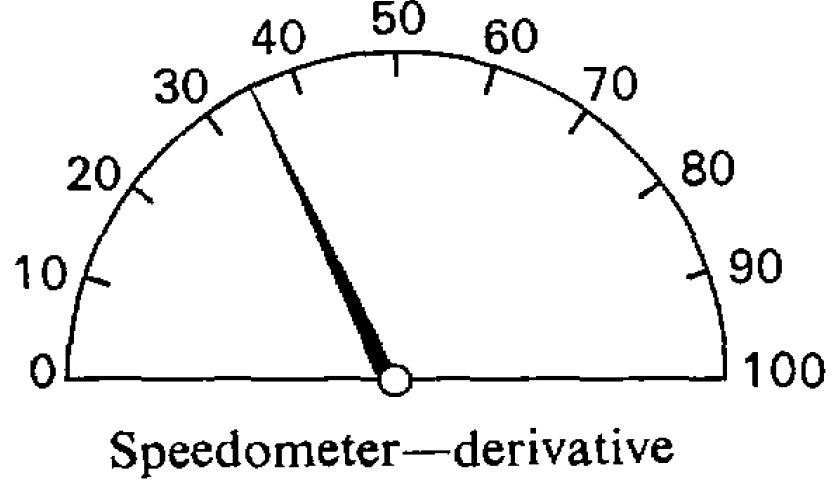Calculating rate of change for simple models is commonsenseCalculating rate of change of a car 

Calculating rate of change is the first of calculus’s two principal operations

…the two principal symbols that are used in calculating… are:

1. d which merely means “a little bit of.”
Thus dx means a little bit of x; or du means a little bit of u.
2. ∫ which is merely a long S, and may be called (if you like) “the sum of.”
Thus ∫ dx means the sum of all the little bits of x… That’s all.

In ordinary algebra which you learned at school, you were always hunting after some unknown quantity which you called x or y; or sometimes there were two unknown quantities to be hunted for simultaneously. You have now to learn to go hunting in a new way; the fox being now neither x nor y. Instead of this you have to hunt for this curious cub called dy/dx. The process of finding the value of dy/dx is called “differentiating.” But, remember, what is wanted is the value of this ratio when both dy and dx are themselves indefinitely small.

Calculating rate of change means calculating a curve’s local slope

It is useful to consider what geometrical meaning can be given to the differential coeffcient. In the first place, any function of x, such, for example, as x2, or √x, or ax + b, can be plotted as a curve…

Consider any point Q on this curve, where the abscissa of the point is x and its ordinate is y. Now observe how y changes when x is varied. If x is made to increase by a small increment dx, to the right, it will be observed that y also (in this particular curve) increases by a small increment dy (because this particular curve happens to be an ascending curve). Then the ratio of dy to dx is a measure of the degree to which the curve is sloping up between the two points Q and T. If… Q and T are so near each other that the small portion QT of the curve is practically straight, then it is true to say that the ratio dy/dx is the slope of the curve along QT.

Calculating rate of change just takes a little algebra and common sense

Now let us see how, on first principles, we can differentiate some simple algebraical expression. Let us begin with the simple expression y = x2. Let x, then, grow a little bit bigger and become x + dx; similarly, y will grow a bit bigger and will become y + dy. Then, clearly, it will still be true that the enlarged y will be equal to the square of the enlarged x. Writing this down, we have:

y + dy = (x + dx)2.

Doing the squaring we get:

y + dy = x2 + 2x∙dx + (dx)2.

Remember that dx meant a bit—a little bit—of x. Then (dx)2 will mean a little bit of a little bit of x; that is… it is a small quantity of the second order of smallness. It may therefore be discarded as quite inconsiderable in comparison with the other terms. Leaving it out, we then have:

y + dy = x2 + 2x∙dx.

Now y = x2; so let us subtract this from the equation and we have left

dy = 2x∙dx.

Dividing across by dx, we find

dy/dx= 2x.

Now this is what we set out to find. The ratio of the growing of y to the growing of x is, in the case before us, found to be 2x.

1. Keisler, H. Jerome. Elementary calculus: An infinitesimal approach. Courier Corporation, 2012, p. xi.
2. Thompson, Silvanus Phillips. Calculus made easy. 2nd ed., enlarged, MacMillan, 1914.